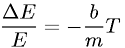Equations > Physics > Oscillations and Waves > Energy change in a damped oscillation

### Energy change in a damped oscillationLatex Code:

MathML Code:

 $\frac\Delta EE=-\fracbmT$

MathType 5.0: